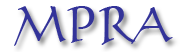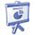Home

Estimating multivariate GARCH and Stochastic Correlation models equation by equation

Christian Francq and Jean-Michel Zakoïan
Keywords: Constant conditional correlation, Dynamic conditional correlation, Markov switch- ing correlation matrix, Multivariate GARCH specification testing, Quasi maximum likelihood estimation.
Abstract: This paper investigates the estimation of a wide class of multivariate volatility mod- els. Instead of estimating a m-multivariate volatility model, a much simpler and numerically efficient method consists in estimating m univariate GARCH-type models Equation by Equation (EbE) in the first step, and a correlation matrix in the second step. Strong consistency and asymptotic normality (CAN) of the EbE estimator are established in a very general framework, including Dynamic Conditional Correlation (DCC) models. The EbE estimator can be used to test the restrictions imposed by a particular MGARCH specification. For general Constant Conditional Correlation (CCC) models, we obtain the CAN of the two-step estimator. Compar- isons with the global method, in which the model parameters are estimated in one step, are provided. Monte-Carlo experiments and applications to financial series illustrate the interest of the approach.
Current version of the paperMPRA working paper
Slides of a talk given atVilnius, July 3, 2014
Archive with the R codes and data sets for the numerical illustrations of the paper(extract the zip file and see the file readme.txt for an explanation of the R codes)